Editor Ratings:
User Ratings:
[Total: 0 Average: 0]

Power Analysis is a statistical tool that is used to estimate the smallest sample size that is required for an experiment. Power analysis greatly helps to figure out if the result of an experiment is genuine and worthwhile or due to chance. It is widely used in domains such as biology, medicine, clinical trials and more.

Determination on sample size is a key statistical study to find out the number of observations or items to be included in a sample. To be relevant, a sample size should be large enough to be capable of depicting differences between various groups or studies, hence the great importance attached to it in the field of statistics.

In this article we will be exploring 5 Free Web based Statistical Power Analysis tools that will be of great help to you to estimate the sample size of your study correctly.

## 1. GigaCalculator – Power and Sample Size Calculator

This is a popular and advanced Statistical Power Calculator that you can use to calculate the required sample size for one-sample statistic or for differences between two proportions in case of two independent samples. The tool is very simple, straightforward, and easy to use but some basic experience with statistical terms is a must in order to use it correctly.

All that you are required to do is input the Sample size, Alpha, Minimum Detectable Effect (MDE), Mean etc and click on Calculate to get the Power for the given sample size.

Additionally, you can scroll down the page and learn more about using the Power and Sample Size calculator including information pertaining to the statistical terms related to calculation and why these statistics calculations are so important in trials and experiments.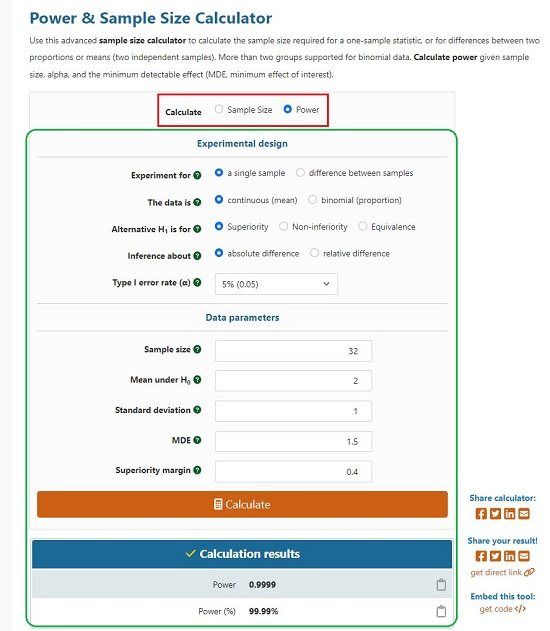## 2. PowerandSampleSize.com

Let’s move on to the next free online tool in this regard namely, powerandsamplesize.com. It has been developed by a bunch of analysts who design experiments and surveys on a regular basis.

The website boasts of a list of 30 calculators related to Power and Sample Size such as Test 1 Mean, Compare 2 Means, Test 1 Proportion, Compare 2 Proportions, Test Odds Ratio and more. You can choose any calculator by clicking on its link in the panel towards the left, provide all the required inputs for the study and then click on ‘Calculate’ to get the results.

Powerandsamplesize.com also provides a very handy and useful visual (graph) representation of the calculation that you can use for further in-depth analysis. Additionally, you can scroll down the page to learn more about the calculation in question as well as the related formulae.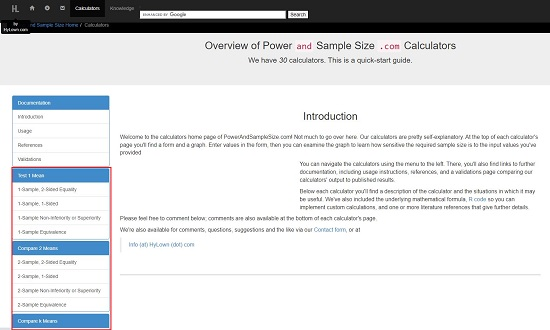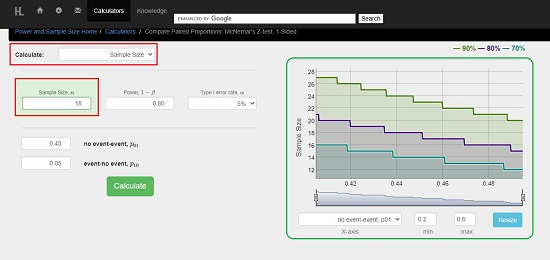## 3. Free Statistics Calculators

The next website that we will be exploring is Free Statistics Calculators which boasts of a range of calculators including Power and Sample Size calculators that can be freely used by scientists, students, researchers, or any other interested persons.

Just click on the link for the calculator – Statistical Power (or Sample Size), feed the required inputs and click on ‘Calculate’ to get the results instantly. As in the case of the above listed tools, this website too provides additional information about the calculation being performed including the formulae.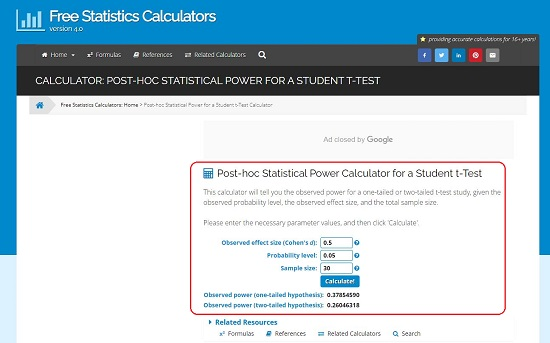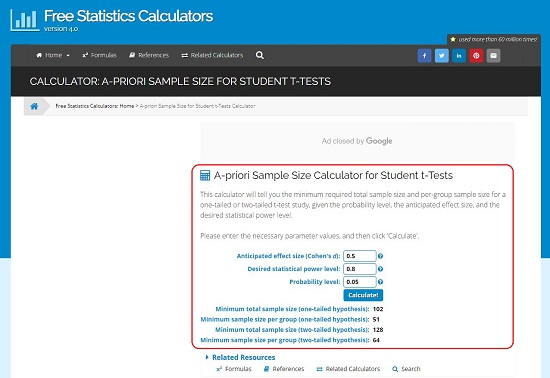## 4. Statistics Kingdom

This is yet another widely used tool for Statistical Power and Sample Size calculations along with a host of other calculators as the name suggests.

The working is similar to the previous tools that we have explored above. Just click on the required calculator such as Z Test power, T Test power, F Test power, Regression power etc. and provide all the required inputs for the calculations. The inputs for calculation can be fed directly to the website using the input boxes or by way of Raw or Excel data. Click on the corresponding buttons if you wish to input the data using the latter method.

Now, click on ‘Calculate test’ to get the results instantly along with a graphic representation of the distribution and interpretation of the result.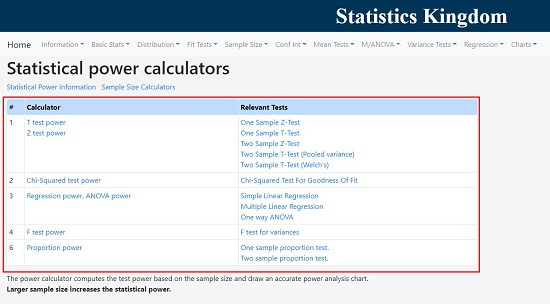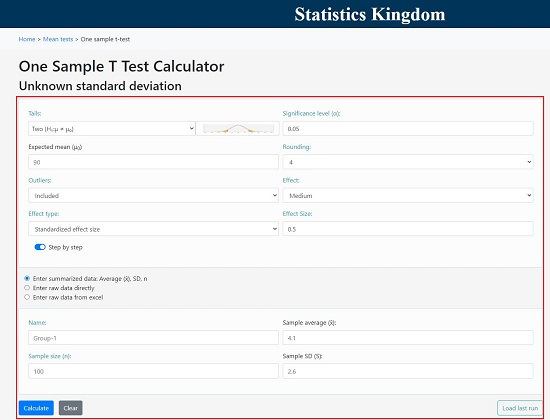## 5. AI Therapy Statistics

Let’s now move to the last Statistical Power tool namely AI Therapy Statistics. Like few of the above tools that we have explored, this website too hosts a range of Statistical calculators including Power and Sample Size.

The working remains the same as we have discussed for the other tools. Simply choose the Power Calculator that you require such as T Test, F Test etc, feed all the required inputs and click on ‘Submit’ to get the results. For Sample Size calculation, click on the corresponding link in the panel towards the left and repeat the same procedure to perform the analysis.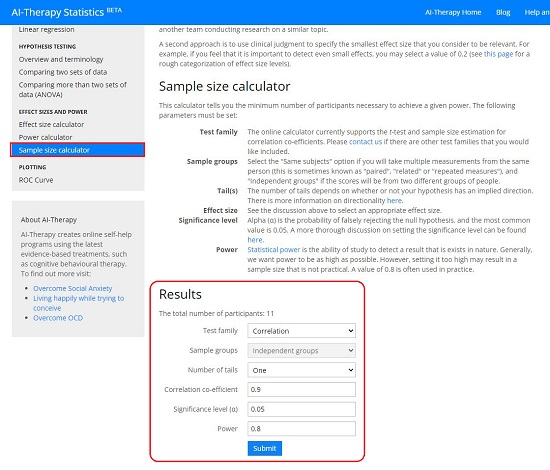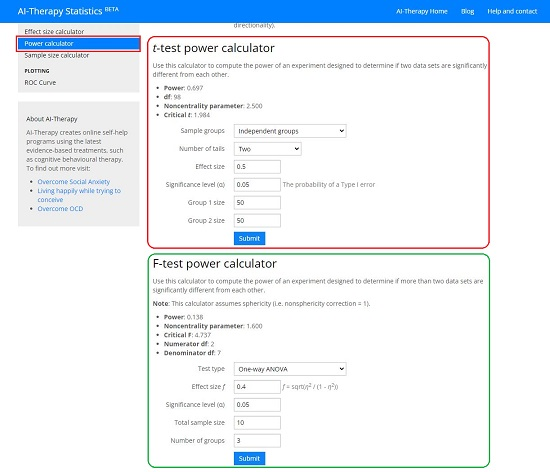### Closing Words:

You can use any of the above listed tools for your Statistical Power or Sample Size calculation. Try to spend some time with each website and figure out which one of them best suits your requirements. Statistics Kingdom and PowerandSample Size.com help you with a graphical visual representation and interpretation of the results, hence they will be my personal choice in this regard. Let us know what are your observations and opinions.

 Editor Ratings: User Ratings:[Total: 0 Average: 0] Free/Paid: Free